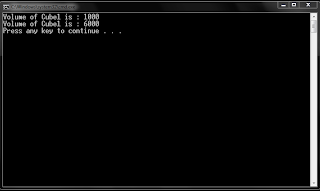Tuesday, 26 August 2014

//Program for Method Overriding
class Bank
{
int rate_of_interest()
{
return 0;
}
}

class SBI extends Bank
{
//override
int rate_of_interest()
{
return 8;
}
}

class ICICI extends Bank
{
//override
int rate_of_interest()
{
return 7;
}
}

class AXIS extends Bank
{
//override
int rate_of_interest()
{
return 9;
}
}

public class Method_Overriding
{
public static void main(String soham[])
{
SBI sbi = new SBI();

ICICI icici = new ICICI();

AXIS axis = new AXIS();

System.out.print("\nSBI Rate of Interest: " + sbi.rate_of_interest());

System.out.print("\nICICI Rate of Interest: " + icici.rate_of_interest());

System.out.print("\nAXIS Rate of Interest: " + axis.rate_of_interest());

System.out.println();
}
}
Outputclass Cube
{
int length;
int height;

Cube()                                                            //constructor 1
{
length = 10;
height = 10;
}

Cube(int length, int breadth, int height)   //constructor 2
{
this.length = length;
this.height = height;
}

public int get_volume()
{
return (length * breadth * height);
}
}

public class Constructor_Demo
{
public static void main(String soham[]) throws Exception
{
Cube cube_obj1 = new Cube();
Cube cube_obj2 = new Cube(10, 20, 30);
System.out.println("Volume of Cube1 is : " + cube_obj1.get_volume());
System.out.println("Volume of Cube1 is : " + cube_obj2.get_volume());
}
}
Output//Program for Scanner Class
import java.util.Scanner;
public class Scanner_Demo
{
public static void main(String soham[]) throws Exception
{
Scanner sc = new Scanner(System.in);

int rollno = sc.nextInt();

String name = sc.next();

double fee = sc.nextDouble();

System.out.print("\nStudents Details Are: ");
System.out.print("\nRoll no: "+rollno);
System.out.print("\nName: "+name);
System.out.print("\nFee: "+fee);

System.out.println();
}
}
Output1.2.